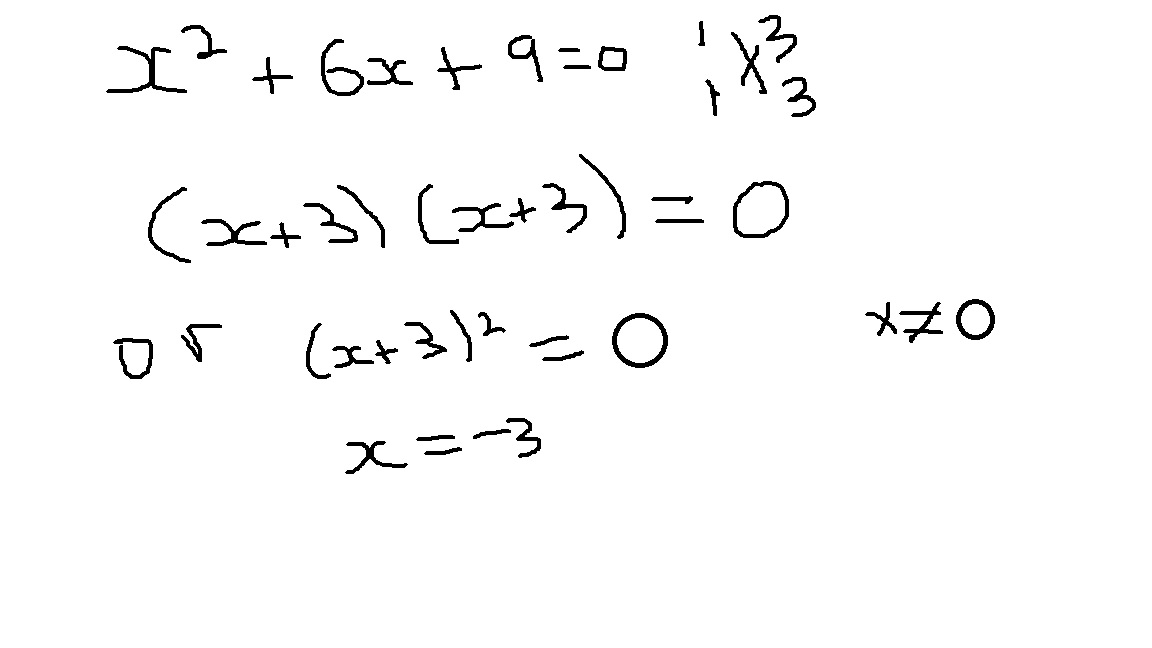# How do you factor the trinomial x^2+6x+9=0?

How do you factor the trinomial x^2+6x+9=0?

ReportAnswer 1

${\left(x + 3\right)}^{2}$=0
so, x=-3

#### Explanation:this is the explanation

##### Add Answer of: How do you factor the trinomial x^2+6x+9=0?
Similar Homework Help Questions

• ### How do you factor the trinomial x^2+3x-36=0#?

Need Online Homework Help?